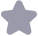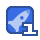### 数字信号处理西安电子高西全课后习题答案收藏

Tax1(n)]=ax()+2ax1(-1)+3ax1(n-2) 7bx2(m)=bx2()+2bx2(n-1)+3bx2(n-2) TAx, (n)+bx, (n]=aTIx, (n)]+bTIx,(n) 故该系统是线性系统。 (3)这是一个延时器,延时器是一个线性时不变系统,下面予以证 明 令输入为x(n-n1),输出为y(m)=xn-n1-n),因为 n1)=x(n-n1-1)=y 故延时器是一个时不变系统。又因为 Tlax, (n)+bx,(n)]=ax, (n-no)+bx,(n-no)=aTIx(n)]+brIx, (n)] 故延时器是线性系统。 (5) V(n 令:输入为x(n-n),输出为y(n)=x2(n-n),因为 (n-n0)=x(n-n0)=y(m) 故系统是时不变系统。又因为 FLax (n)+bx, (n=(ax, (n)+bx, (n)) TIx(n+bRIx, (n) xx,(n)+bx,(n) 因此系统是非线性系统。 (7) y(n) x 令:输入为x(n-n),输出为y(n)=∑x(m-n),因为 y(n-n)=∑x(m)≠y(n) 故该系统是时变系统。又因为 Flax, (n)+bx, (n)] ∑ (ax (m)+bx, (m)=aTIx, (n)]+bTLx, (n) 用=0 故系统是线性系统 6.给定下述系统的差分方程,试判断系统是否是因果稳定系统,并 说明理由。 (1)y(n)=1∑xn-k); (3)y(n)=∑x(k); = (5)y(m)=em)。 解 (1)只要N21,该系统就是因果系统,因为输出只与n时刻的和n 时刻以前的输入有关。如果xn)≤M,则ym≤M,因此系统是稳定 系统。 (3)如果(o)≤M,o0∑xk)2n+M,因此系统是稳定的。 kan-no 系统是非因果的,因为输出还和x(n)的将来值有关 (5)系统是因果系统,因为系统的输出不取决于x(n的未来值。如 果x()≤M,则y(m)=-lcse",因此系统是稳定的。 7.设线性时不变系统的单位脉冲响应h(n)和输入序列x(m)如题7图所 示,要求画出输出输出y(n)的波形。 解 解法(1):采用图解法 x(n)*h(n)=>x(m)h(n-m) 图解法的过程如题7解图所示。 解法(2):采用解析法。按照题7图写出x(n)和h(n)的表达式: x(n)=-6(m+2)+(m-1)+26(n-3) (n)=26(m)+6(n-1)+-O(n-2) 因为 x(n)8和=)x的) )*A H A tn y(n)=x(n)*[26(m)+6(n-1)+6(n-2) 所以 2x(n)+x(n-1)+=x(n-2) 将x(n)的表达式代入上式,得到 y(n)=-26(n+2)-6(n+1)-0.56(m)+26(n-1)+(n-2) +4.56(n-3)+26(n-4)+(n-5) 8.设线性时不变系统的单位取样响应h(n)和输入x(n)分别有以下三 种情况,分别求出输出y(n)。 (1)h(n)=R1(n),x(n)=R(n); (2)h)=2R(n),x(m)=m)-(n-2); (3)h(n)=0.5u(n),xn=R(m) 解: h(n)=∑R(m)R(n-m) 先确定求和域,由R(m)和R(n-m)确定对于m的非零区间如下: 0≤m≤3,n-4≤m≤n 根据非零区间,将n分成四种情况求解: ①n<0,y(n)=0 ②20≤ns3,y(n)=∑1=n+1 =0 4sns7,ym)=∑1= =n-4 ④7<n,y(n)=0 最后结果为 n<0,n>7 y(n)={n+1,0≤n≤3 4≤n≤7 yn)的波形如题8解图(一)所示。 (2) y(n)=2R4(m)*[o(n)-6(n-2)=2R(n)-2R2(n-2) =2|(m)+(n-1)-6(n-4)-(n-5) y(n)的波形如题8解图(二)所示 (3) )*h(n) =∑R(m0.5(n-m)=05∑Rm05"u(n-m) =0 ==O0 y(n)对于m的非零区间为0≤m≤4,m≤n。 ①n<0,y(n)=0 ②0≤ns4y0)0505=105-05=4-05)05=2-05 0.5 ③55n,y(n)=05∑05m 1-0.55 0.5″=31×0.5 1-0.5 最后写成统一表达式: y(n)=(2-0.5")R(n)+31×0.5u(n-5 11.设系统由下面差分方程描述 y(n)=y(n-1)+x(n)+=x(n-1); 2 设系统是因果的,利用递推法求系统的单位取样响应。 解: :x(n)=(n) h(n)==h(n-1)+o(n)+(n-1) n=0,h(O)=h(-1)+6(0)+(-1)=1 n=1,h(1)=-h(0)+6(1)+6(0)=1 n=2,h(2)=-h(1)= n=3,h3)≈1 h(2) 归纳起来,结果为 h(n)=( 2(n-1)+6(n) 12.有一连续信号x()=cos(2nn+o,式中,f=20H,=2 (1)求出x()的周期。 (2)用采样间隔T=002s对x()进行采样,试写出采样信号元()的表 达式 (3)画出对应(n)的时域离散信号(序列)x(n)的波形,并求出x(n)的 周期 第二章 教材第二章习题解答 1.设X(e")和Y(e~)分别是xmn)和y(n)的傅里叶变换,试求下面序列的 傅里叶变换: (1)x(n-n); (2)x(-m); (3)x(n)y(m); (4)x(2n)。 解 (1)FTIx(n-no)=2x(n-noDe /m n n-no, n=n +no FTIx(n-no)]=2 x(n )e n +m)=e mX(e") (2)Fx(m=∑x(n)e=I∑xnle-"=x(e-) (3)Fx(-m)=∑ -ne 令n=-n,则 Fx(-n)=∑xn)e=X(e") (4) FTlr(n)y(n)=X(er(e) 证明 x(n)*y(n)= ∑ x(m)y(n-m) Fx(n)*y=∑[∑x(m)y(m 令 k=n- 则 FLx(n)*y(m)=∑∑x(m)yk)ee ∑y(k)e∑xm)e X(e/)Y(e/) w< w 2.已知x(e-)= 0,w<w≤r 求X(e-)的傅里叶反变换x(n)。 解: e/ dv sIn w 2丌 n 3.线性时不变系统的频率响应(传输函数)H(")=|H(e)e,如果单 位脉冲响应hm)为实序列,试证明输入x(n)=Acos(mn+)的稳态响应 为 [won+o+e(wo)J 解: 假设输入信号x(m)=e~,系统单位脉冲相应为h(n),系统输出为 y(n)=h(n)*x(n)= ∑ h(me ∑h(m)emm=H(e)e 上式说明,当输入信号为复指数序列时,输出序列仍是复指数序列, 且频率相同,但幅度和相位决定于网络传输函数,利用该性质解此题。 x(n)=Acos(wo/+)=A[e/e9+e "e/91 y(n)=A[ pe/"H(e o)+"e o"H(e o ) 2 Alee/H(e "o)e/e( o) +e" e no H(e" m )e/o-wl 上式中H(e)是w的偶函数,相位函数是w的奇函数, e H(e),O(w)=-b(-w) (n)=AH(eo)le +e-e A H(e" o )cos(w/+9+ 0 (w) l,n=0,1 4.设xn)=30其它 将x(n)以4为周期进行周期延拓,形成周期序列 x(m),画出x(n)和x(m)的波形,求出x(n)的离散傅里叶级数x(k)和傅 里叶变换。 解 画出x(n)和(m)的波形如题4解图所示。 X(k)=DFS[(m)=∑(n) ∑ I+e e4(e4+e4)=2cos(k)·e 8(k)以4为周期,或者 sin -Tk X(k)=∑ e e j-k sin-丌k X(k)以4为周期 X(e/)=FTIi(n)]=2X()S(w-k) ∑ X(k6(w--k) r∑cos(akb(w-k) 5.设如图所示的序列x(n)的FT用x(e-)表示,不直接求出X(e-), 完成下列运算: (1)X(e); (2)[x

...展开详情

抢沙发
一个资源只可评论一次，评论内容不能少于5个字
qq_33766759关注 私信 TA的资源
上传资源赚积分,得勋章
最新推荐数字信号处理西安电子高西全课后习题答案 9积分/C币 立即下载
1/44试读已结束，剩余30页未读...

9积分/C币 立即下载 ＞